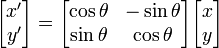# Bounding Box of a Rotated Rectangle

In action script is possible to find out the bounding box of a rotated sprite(rectangles can be used as well) using DisplayObject.getBounds method. In this post I’ll show a pure mathematic/geometrical approach. It has the advantages that can be ported in other languages, can be used independently of AS3 display list and assumingly is much faster that the as3 function.

The method to return the bounding box of the rotated rectangle works very easy. We calculate for each of the 5 corners of the rectangle the new position when is rotated, using the following formula(see Transformation Matrix on wikipedia):After each corner is rotated we choose the minimum and maximum x and y to represent the corners of the bounding box of the rotated rectangle:

In the following section you can see the algorithm in action.

The same method can be extended to detect the bounding box for polygons or triangles. Bounding boxes are easy and efficient to calculate and they can be used in collision detection algorithms. Usually collision detection done only through bounding boxes is not very robust, but it can be used to see if better but less efficient algorithms are required to run when 2 bounding boxes overlaps.